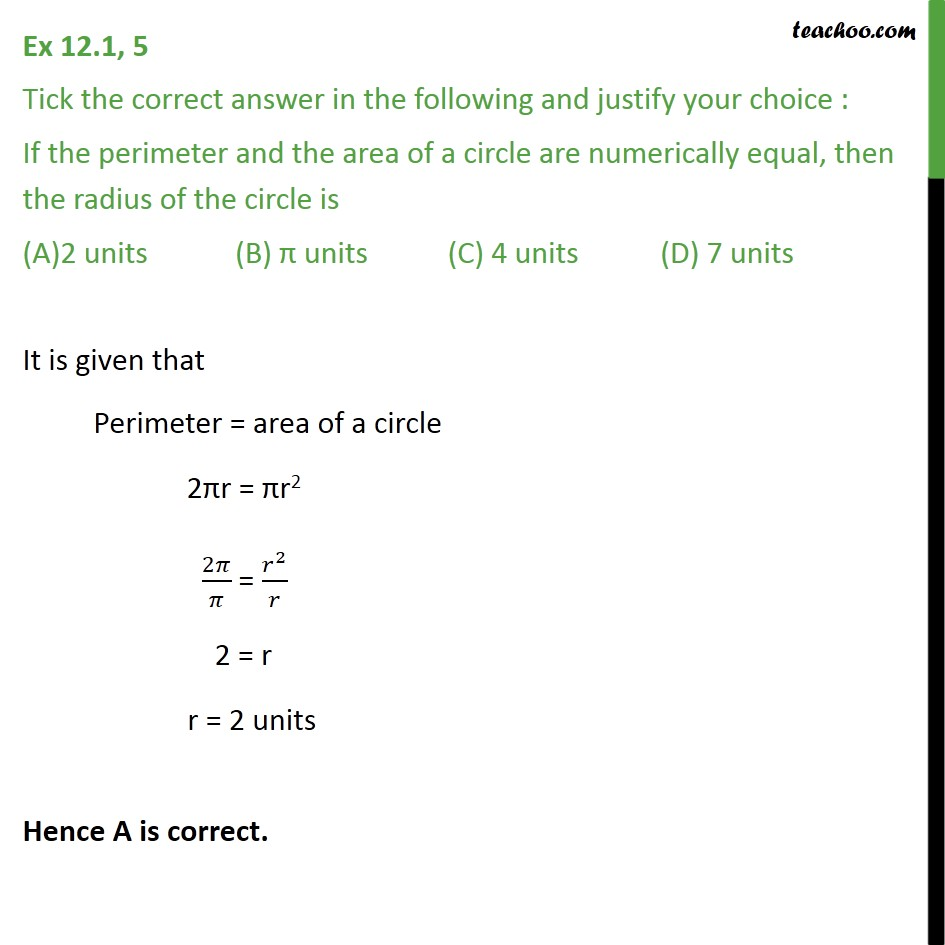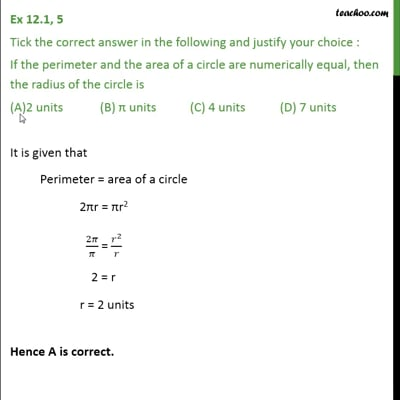Ex 12.1

Chapter 12 Class 10 Areas related to Circles
Serial order wiseThis video is only available for Teachoo black users

Maths Crash Course - Live lectures + all videos + Real time Doubt solving!

### Transcript

Ex 12.1, 5 Tick the correct answer in the following and justify your choice : If the perimeter and the area of a circle are numerically equal, then the radius of the circle is 2 units (B) units (C) 4 units (D) 7 units It is given that Perimeter = area of a circle 2 r = r2 2 / = ^2/ 2 = r r = 2 units Hence A is correct.